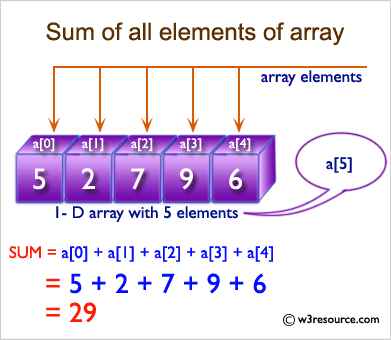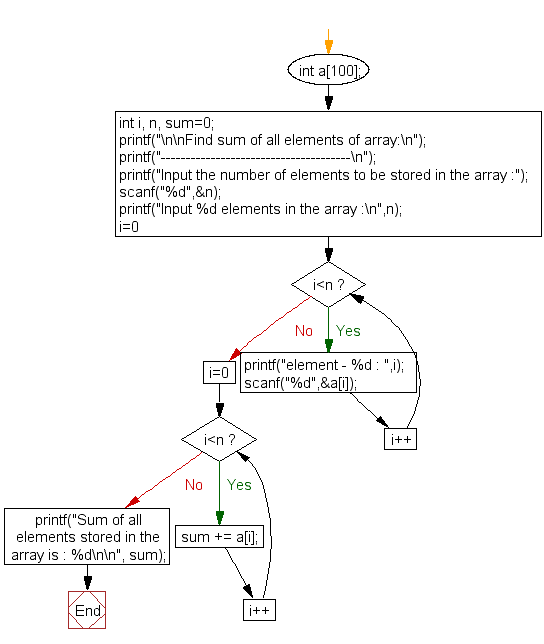﻿ C Program: Find the sum of all elements of an array - w3resource

# C Exercises: Find the sum of all elements of an array

## C Array: Exercise-3 with Solution

Write a program in C to find the sum of all elements of an array.

Visual Presentation:Sample Solution:

``````#include <stdio.h>
// Main function
int main()
{
int a;  // Declare an array of size 100 to store integer values
int i, n, sum = 0;  // Declare variables to store array size, loop counter, and sum
// Display a message to the user about the program's purpose
printf("\n\nFind sum of all elements of array:\n");
printf("--------------------------------------\n");
// Prompt the user to input the number of elements to be stored in the array
printf("Input the number of elements to be stored in the array :");
scanf("%d", &n);
// Prompt the user to input n elements into the array
printf("Input %d elements in the array :\n", n);
for (i = 0; i < n; i++)
{
printf("element - %d : ", i);
scanf("%d", &a[i]);  // Read the input and store it in the array
}

// Calculate the sum of all elements in the array using a for loop
for (i = 0; i < n; i++)
{
sum += a[i];  // Add each element to the sum
}
// Display the sum of all elements stored in the array
printf("Sum of all elements stored in the array is : %d\n\n", sum);
return 0;
}
```
```

Sample Output:

```Find sum of all elements of array:
--------------------------------------
Input the number of elements to be stored in the array :3
Input 3 elements in the array :
element - 0 : 2
element - 1 : 5
element - 2 : 8
Sum of all elements stored in the array is : 15
```

Explanation:

```  printf("Input the number of elements to be stored in the array :");
scanf("%d",&n);

printf("Input %d elements in the array :\n",n);
for(i=0;i<n;i++)
{
printf("element - %d : ",i);
scanf("%d",&a[i]);
}

for(i=0; i<n; i++)
{
sum += a[i];
}

printf("Sum of all elements stored in the array is : %d\n\n", sum);
```

In the above code -

• The first printf statement prompts the user to input the number of elements they want to store in the array and stores it in the variable n using scanf.
• The second printf statement asks the user to input n number of elements into the array using a for loop, and stores each input in the corresponding index of the array a[i].
• The third for loop calculates the sum of all the elements in the array by iterating over each element of the array and adding its value to the variable sum.
• The final printf statement then prints out the sum of all the elements in the array using the value stored in the variable sum.

Flowchart :C Programming Code Editor:

What is the difficulty level of this exercise?

Test your Programming skills with w3resource's quiz.

﻿